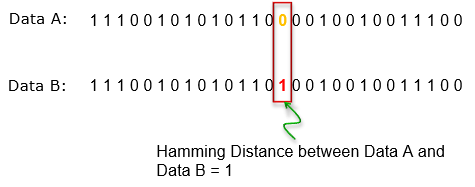# Hamming Distance Calculation using Python

While writing for my master I wrote a series of codes in python to achieve what I wanted, such asslicing the video in small frames, generate the hash for each image and compare the hamming distance of each hach. Here is the piece where I compare the two values and generate the hamming distance of it.

Hamming Distance – Is a metric for comparing two binary data strings. While comparing two binary strings of equal length, Hamming distance is the number of bit positions in which the two bits are different.Hamming Distance

The code compares the values at a byte level and I added a function that transforms the received hash value into a binary value

```#!/usr/bin/python
import binascii
class hamming :
def __ init __( self ):
pass

def transform (self , hexvalue ):
integer = int ( hexvalue ,16)
binvalue = format ( integer ,’0 >64b’)
return binvalue

def distance (self ,a,b):
lengthofa = len (a)
lengthofb = len (b)
if lengthofa != lengthofb :
return False
count = 0
for i in range (0, lengthofa ):
if a[i] != b[i]:
count += 1
return count
```

Usage

```#!/usr/bin/python
from hamming . hamming import hamming
h = hamming()
distance = h.distance(h.transform(VALUE1),h.transform(VALUE2))
print distance
```